# Parts of a cathode ray tube The filament

• Slides: 9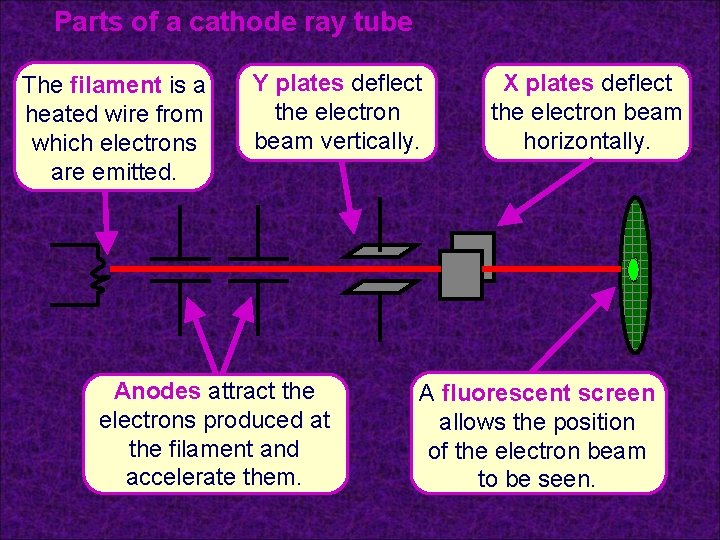Parts of a cathode ray tube The filament is a heated wire from which electrons are emitted. Y plates deflect the electron beam vertically. Anodes attract the electrons produced at the filament and accelerate them. X plates deflect the electron beam horizontally. A fluorescent screen allows the position of the electron beam to be seen.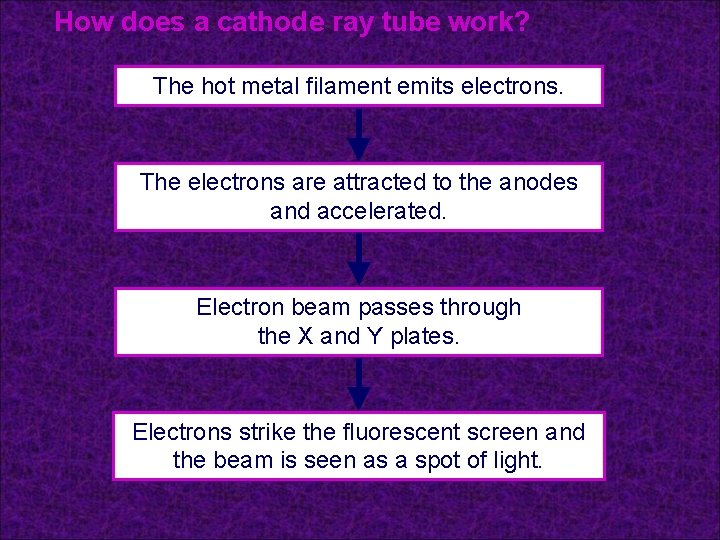How does a cathode ray tube work? The hot metal filament emits electrons. The electrons are attracted to the anodes and accelerated. Electron beam passes through the X and Y plates. Electrons strike the fluorescent screen and the beam is seen as a spot of light.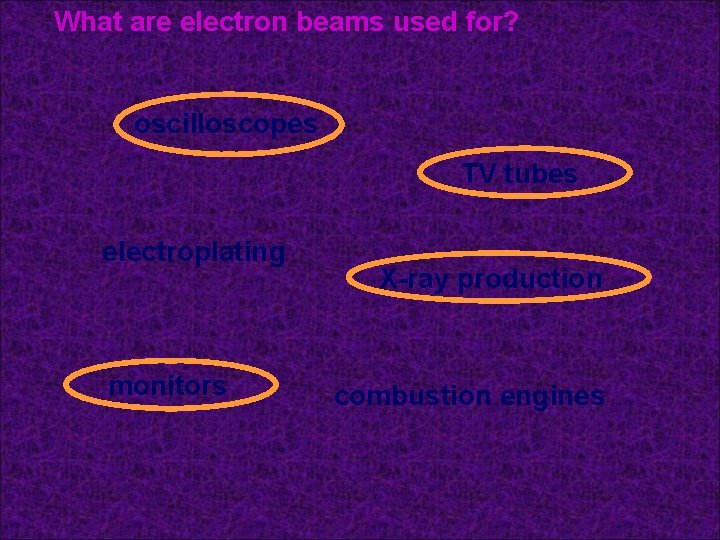What are electron beams used for? oscilloscopes TV tubes electroplating monitors X-ray production combustion engines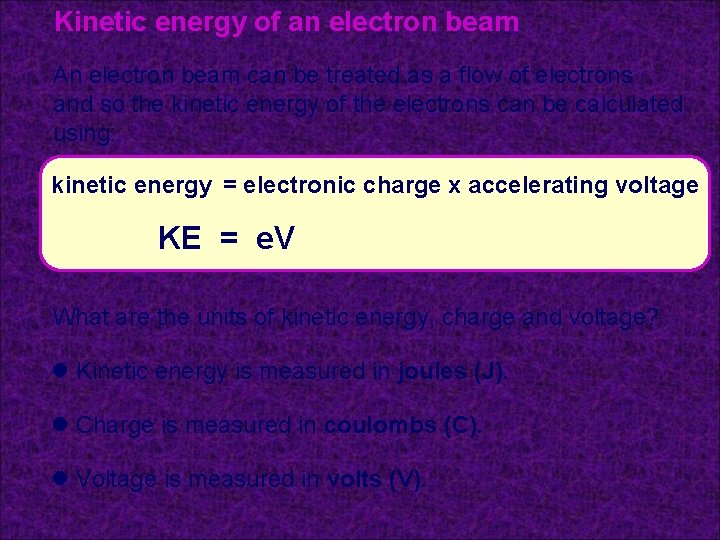Kinetic energy of an electron beam An electron beam can be treated as a flow of electrons and so the kinetic energy of the electrons can be calculated using: kinetic energy = electronic charge x accelerating voltage KE = e. V What are the units of kinetic energy, charge and voltage? l Kinetic energy is measured in joules (J). l Charge is measured in coulombs (C). l Voltage is measured in volts (V).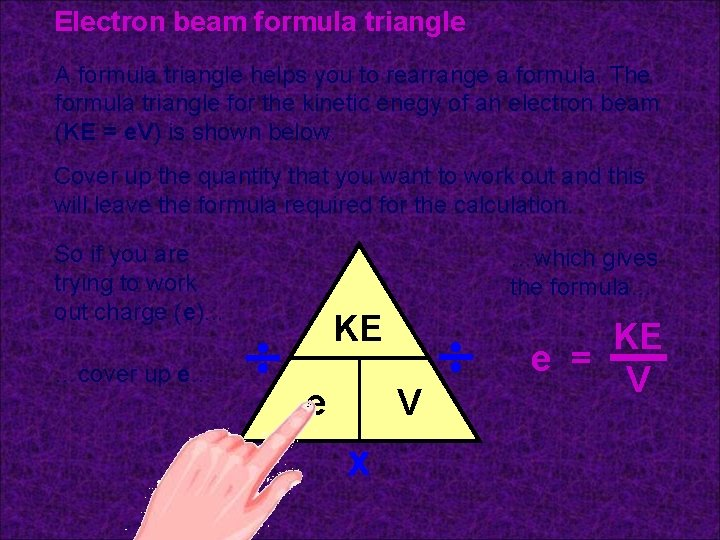Electron beam formula triangle A formula triangle helps you to rearrange a formula. The formula triangle for the kinetic enegy of an electron beam (KE = e. V) is shown below. Cover up the quantity that you want to work out and this will leave the formula required for the calculation. So if you are trying to work out charge (e). . . …cover up e… …which gives the formula… KE e V x KE e = V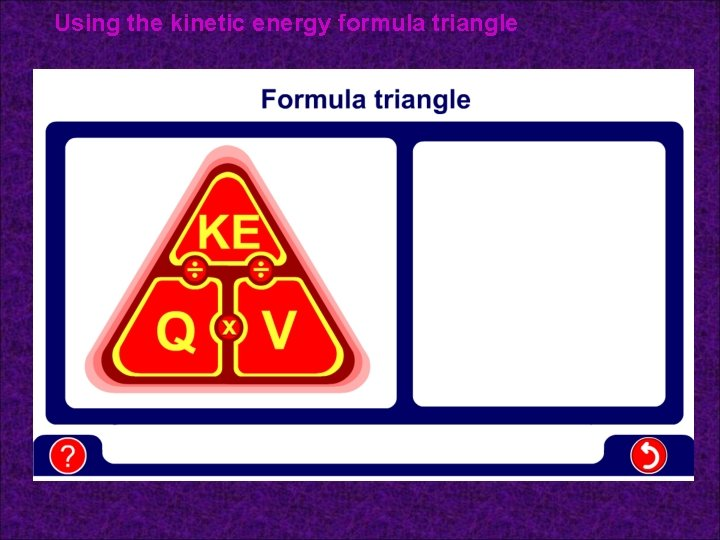Using the kinetic energy formula triangle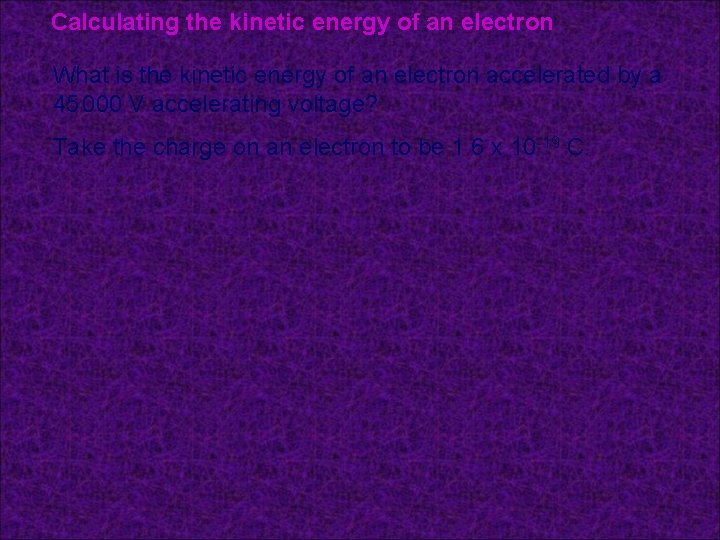Calculating the kinetic energy of an electron What is the kinetic energy of an electron accelerated by a 45 000 V accelerating voltage? Take the charge on an electron to be 1. 6 x 10 -19 C.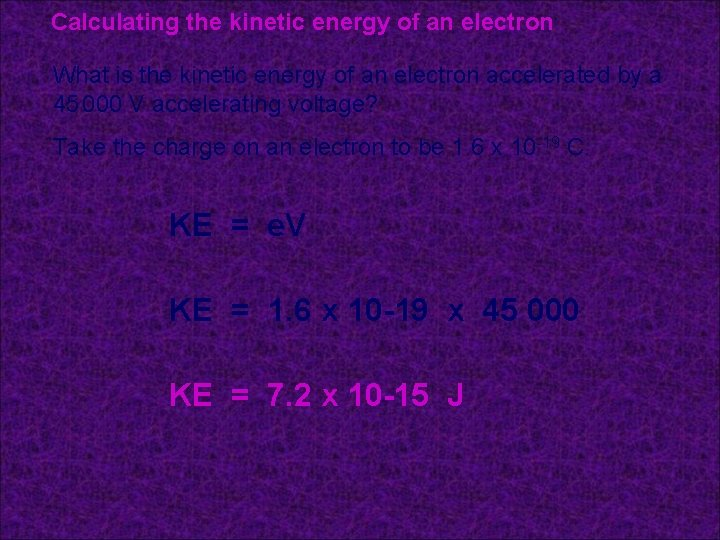Calculating the kinetic energy of an electron What is the kinetic energy of an electron accelerated by a 45 000 V accelerating voltage? Take the charge on an electron to be 1. 6 x 10 -19 C. KE = e. V KE = 1. 6 x 10 -19 x 45 000 KE = 7. 2 x 10 -15 JGlossary l absolute zero – The lowest possible temperature which is equal to -273°C. At this temperature particles cannot move any slower and have the minimum kinetic energy. l cathode ray tube – A device with a heated filament which emits an electron beam that can be seen when it hits a fluorescent screen. l Einstein – The scientist who worked out how to calculate the energy released when mass changes into energy. l electron beam – A stream of electrons that have been emitted from a hot cathode and accelerated by anodes. l Kelvin – The temperature scale which uses absolute zero as its zero (0 K). l pressure – This property of a gas is caused its particles bouncing off the walls of the container. It is directly proportional to the temperature of the gas.